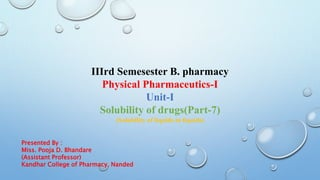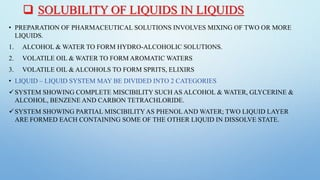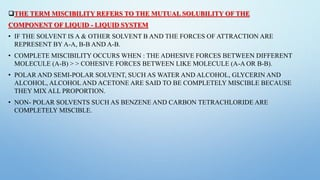Diese Präsentation wurde erfolgreich gemeldet.

# Solubility of liquds in liquids

Anzeige
Anzeige
Anzeige
Anzeige
Anzeige
Anzeige
Anzeige
Anzeige
Anzeige
Anzeige
Anzeige×

1 von 12 Anzeige

# Solubility of liquds in liquids

Solubility of liquids in liquids, The term miscibility refers to the mutual solubility of the component of liquid - liquid system, Raoult’s Law, Raoult’s law may be mathematically expressed as: Ideal solution, Real solution

Solubility of liquids in liquids, The term miscibility refers to the mutual solubility of the component of liquid - liquid system, Raoult’s Law, Raoult’s law may be mathematically expressed as: Ideal solution, Real solution

Anzeige
Anzeige

### Solubility of liquds in liquids

1. 1. IIIrd Semesester B. pharmacy Physical Pharmaceutics-I Unit-I Solubility of drugs(Part-7) (Solubility of liquids in liquids) Presented By : Miss. Pooja D. Bhandare (Assistant Professor) Kandhar College of Pharmacy, Nanded
2. 2.  SOLUBILITY OF LIQUIDS IN LIQUIDS • PREPARATION OF PHARMACEUTICAL SOLUTIONS INVOLVES MIXING OF TWO OR MORE LIQUIDS. 1. ALCOHOL & WATER TO FORM HYDRO-ALCOHOLIC SOLUTIONS. 2. VOLATILE OIL & WATER TO FORM AROMATIC WATERS 3. VOLATILE OIL & ALCOHOLS TO FORM SPRITS, ELIXIRS • LIQUID – LIQUID SYSTEM MAY BE DIVIDED INTO 2 CATEGORIES SYSTEM SHOWING COMPLETE MISCIBILITY SUCH AS ALCOHOL & WATER, GLYCERINE & ALCOHOL, BENZENE AND CARBON TETRACHLORIDE. SYSTEM SHOWING PARTIAL MISCIBILITYAS PHENOLAND WATER; TWO LIQUID LAYER ARE FORMED EACH CONTAINING SOME OF THE OTHER LIQUID IN DISSOLVE STATE.
3. 3. THE TERM MISCIBILITY REFERS TO THE MUTUAL SOLUBILITY OF THE COMPONENT OF LIQUID - LIQUID SYSTEM • IF THE SOLVENT IS A & OTHER SOLVENT B AND THE FORCES OF ATTRACTION ARE REPRESENT BY A-A, B-B AND A-B. • COMPLETE MISCIBILITY OCCURS WHEN : THE ADHESIVE FORCES BETWEEN DIFFERENT MOLECULE (A-B) > > COHESIVE FORCES BETWEEN LIKE MOLECULE (A-A OR B-B). • POLAR AND SEMI-POLAR SOLVENT, SUCH AS WATER AND ALCOHOL, GLYCERIN AND ALCOHOL, ALCOHOL AND ACETONE ARE SAID TO BE COMPLETELY MISCIBLE BECAUSE THEY MIX ALL PROPORTION. • NON- POLAR SOLVENTS SUCH AS BENZENE AND CARBON TETRACHLORIDE ARE COMPLETELY MISCIBLE.
4. 4. • BINARY SOLUTION IS A MIXTURE OF TWO LIQUIDS THAT ARE COMPLETELY MISCIBLE ONE WITH ANOTHER. THE BOILING POINT OF BINARY SOLUTION DEPENDS UPON THE SOLUTION COMPOSITION. RAOULT’S LAW • RAOULT’S LAW STATES THAT THE PARTIAL VAPOUR PRESSURE OF EACH VOLATILE CONSTITUENT IS EQUAL TO THE VAPOUR PRESSURE OF THE PURE CONSTITUENT MULTIPLIED BY ITS MOLE FRACTION THE SOLUTION, AT GIVEN TEMPERATURE. • A MIXTURE OF MISCIBLE LIQUIDS AAND B ARE CONSIDERED.
5. 5. • IN THIS MIXTURE LET PARTIAL VAPOUR PRESSURE EXERTED BY LIQUID A = PA KILOPASCALS (KPA) let partial vapour pressure exerted by liquid B = pB (kPa) let partial vapour pressure exerted by pure liquid A = p°A (kPa) let partial vapour pressure exerted by pure liquid B = p°B (kPa) LET MOLE FRACTION CONCENTRATION OF A IN LIQUID = XA let mole fraction concentration of A in liquid = XB
6. 6. • RAOULT’S LAW MAY BE MATHEMATICALLY EXPRESSED AS: Partial vapour pressure Vapour pressure of mole fraction of a liquid pure liquid of liquid PA p°A XA pB p°B XB • Total vapour pressure : PA pB (p°A XA) (p°B XB) • SOME CONCEPT  Total of mole fraction is always 1. IF MOLE FRACTION OF LIQUID ‘A’ IS 0.7 THEN THE MOLE FRACTION OF LIQUID ‘B’ IS 0.3
7. 7. • PA = P°A XA AND PA = P°A XB • THE SOLUTE-SOLVENT FORCES OF ATTRACTION IS A – B = A-A OR B – B • THE ENTHALPY OF MIXING OF TWO COMPONENTS SHOULD BE ZERO, THAT IS, . ΔMIX H=0. THIS SIGNIFIES THAT NO HEAT IS RELEASED OR ABSORBED DURING MIXING OF TWO PURE COMPONENTS TO FORM AN IDEAL SOLUTION. • THE VOLUME OF MIXING IS EQUAL TO ZERO THAT IS, Δ MIXV=0. THIS MEANS THAT TOTAL VOLUME OF SOLUTION IS EXACTLY SAME AS THE SUM OF THE VOLUME OF SOLUTE AND SOLUTION.
8. 8. • PA> P°A XA AND PB> P°B XB , AS THE TOTAL VAPOUR PRESSURE (P°A XA + P°B XB ) IS GREATER THAN WHAT IT SHOULD BE ACCORDING TO RAOULT’S LAW. • THE SOLUTE-SOLVENT FORCES OF ATTRACTION IS WEAKER THAN SOLUTE-SOLUTE AND SOLVENT-SOLVENT INTERACTION THAT IS, A-B < A-A OR B-B. • THE ENTHALPY OF MIXING IS POSITIVE THAT IS ΔMIX H >0 BECAUSE THE HEAT ABSORBED TO FORM NEW MOLECULAR INTERACTION IS LESS THAN THE HEAT RELEASED ON BREAKING OF ORIGINAL MOLECULAR INTERACTION. • THE VOLUME OF MIXING IS POSITIVE THAT IS, ΔMIX V >0 AS THE VOLUME EXPAND
9. 9. IDEAL SOLUTION • AN IDEAL SOLUTION IS DEFINED AS THE SOLUTION IN WHICH NO VOLUME OR TEMPERATURE CHANGE OCCURS ON MIXING OF THE TWO LIQUIDS AND WHICH COMPLETELY OBEY RAOULT’S LAW. REAL SOLUTION • AN REAL SOLUTION IS DEFINES AS THE SOLUTION IN WHICH CHANGE IN VOLUME OR CHANGE IN TEMPERATURE OR BOTH WHILE MIXING OF TWO LIQUIDS AND DO NOT OBEY RAOULT’S LAW
10. 10. THANK YOU!